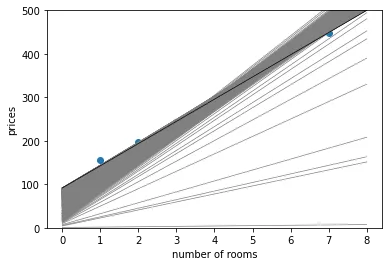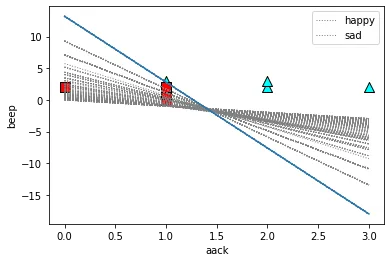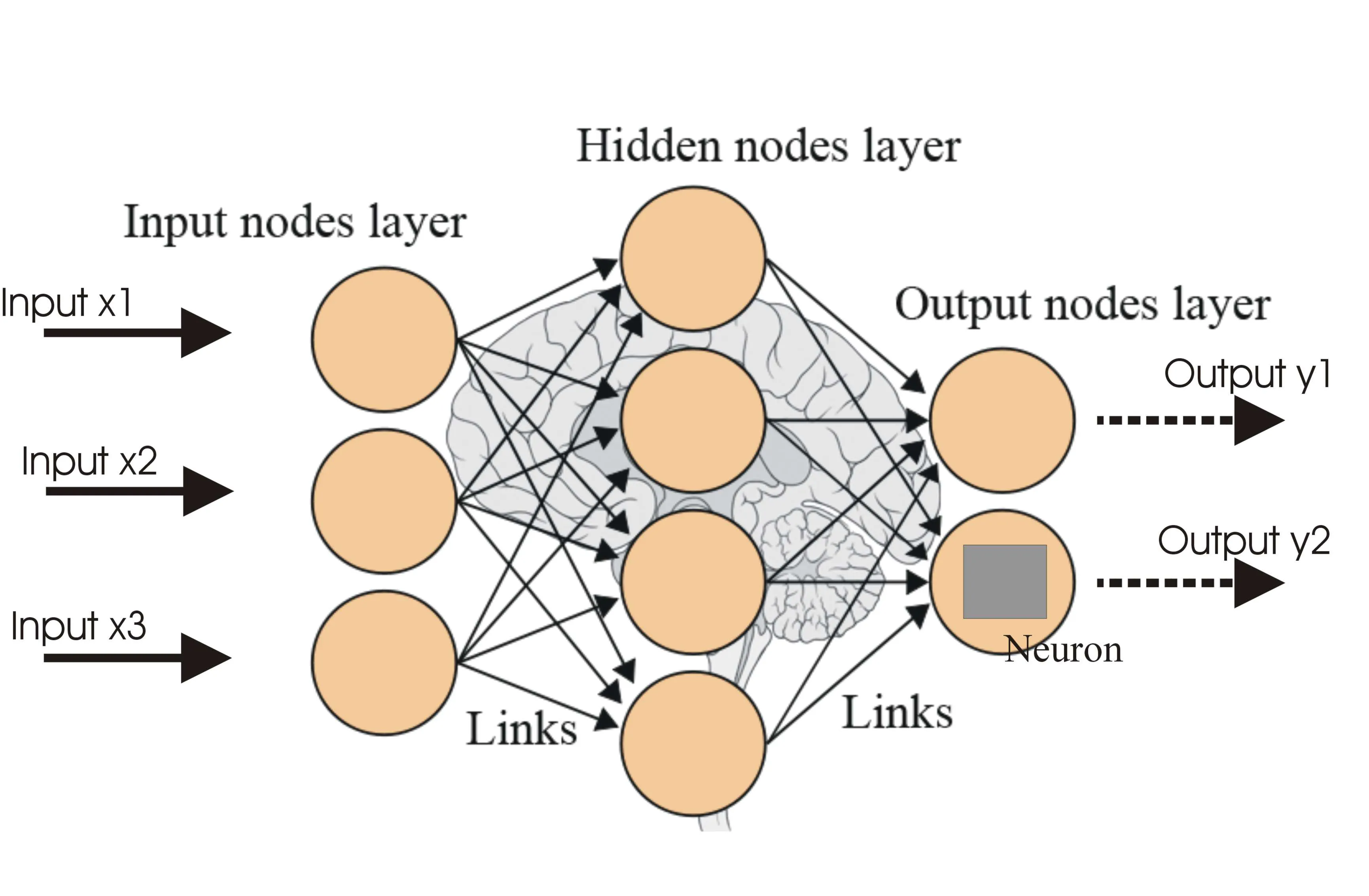# Machine Learning Projects

In God we trust, all others bring data

## Housing Price Detection using Linear Regression

Predicting Numerical Value of a house based on given dataset using Linear Regression

Technologies Used

Python
Numpy
pandas
Matplotlib## Sentiment Analysis using Perceptron Algorithm

Classification Model that analyses the sentiment of a sentence using Perceptron Algorithm

Technologies Used

Python
Numpy
pandas
Matplotlib## Spam Classifier with Naive Bayes

Naive Bayes classifier is a machine learning algorithm that uses statistical techniques to classify input data as either spam or not spam (i.e. ham) based on the probability of certain words or phrases occurring in spam or ham emails.

Python
Numpy
pandas
Tensorflow## Regression Neural Network to predict housing prices

Used a Regression neural network to preduct the housing prices in Hyderabad, India using data collected from Kaggle. Achieved an accuracy of 90%

Python
Numpy
pandas
Tensorflow